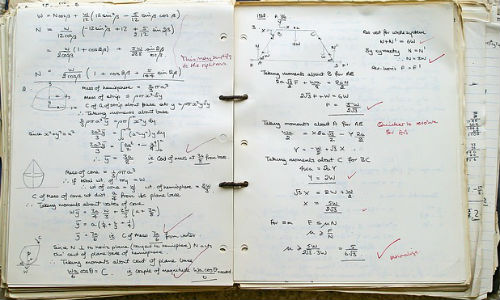@bizjournalismTwo Minute Tips

# Newsroom math crib sheet

September 7, 2017As a journalist, you may not have to deal with math like this, but math is still part of the job. This crib sheet should help. ("School" image by "sandid" via Pixabay, CCO Public Domain)

Arizona State University professor Steve Doig, who won a Pulitzer Prize for his data-based reporting at the Miami Herald, welcomes scores of journalism students to his classroom each year. For the most part, they are much more comfortable with words than numbers. But math is important in the newsroom, and one of Doig’s passions is ensuring that numbers are presented accurately. Regardless if your beat is food or finance, his Newsroom Math Crib Sheet may be the most valuable tool in your reporter’s arsenal. You’re welcome.

## To convert a fraction into a decimal:

• Divide the top number by the bottom number

• Examples: 5/8 = 0.625    17/64 = 0.265

## To convert a decimal into a percentage:

• Multiply by 100 (or simply move the decimal two places to the RIGHT)

• Example: 0.658 = 65.8%

## To turn a percentage into a decimal:

• Divide by 100 (or simply move the decimal two places to the LEFT)

• Example: 148.2% = 1.482

## To get X% of Y:

• Turn X% into a decimal, then multiply it by Y

• Example: 20% of 90 = 0.20 x 90 = 18

## To compare X and Y using percentages (X is what percent of Y?):

• X is (X/Y x 100) percent of Y

• Example: 5 and 8: 5/8 = .625 = 62.5%, so 5 is 62.5% of 8

• Example: 8 and 5: 8/5 = 1.6 = 160%, so 8 is 160% of 5

## To compare X and Y using percentage differences:

• X is ((X/Y –1) x 100) MORE/LESS than Y

• Use MORE THAN if the answer is positive, and LESS THAN if it’s negative

• Example: 5 and 8: 5/8 –1  = .625 – 1 = -0.375 = -37.5%, so 5 is 37.5% less than 8

• Example: 8 and 5: 8/5 –1  = 1.6 – 1 = .6 = 60%, so 8 is 60% more than 5

## To compare a NEW number with an OLD number using percentage change:

• NEW has increased/decreased ((NEW/OLD –1) x 100) percent since OLD

• Or use the formula you learned in middle school: ((new-old)/old) x 100

• Use INCREASED if the answer is positive, and DECREASED if it’s negative

• Example: This year’s \$8 million budget is a 60 percent increase over last year’s \$5 million budget.

• Example: This year’s \$5 million budget is a 37.5 percent decrease from last year’s \$8 million budget.

## To calculate rates (the number of events per some standard unit):

• Do this to account for different size populations

• RATE = (EVENTS / POPULATION ) x (“PER” Unit)

• Example Problem: If there were 320 murders in a population of 1,937,086, what is the murder rate per 100,000?

• First, divide the 320 murders by 1,937,086 = 0.0001652

• Now multiply 0.0001652 x 100,000 = 16.5 murders per 100,000 population

## To calculate the effect of inflation using the Consumer Price Index (CPI):

Price Now   =   CPI Now

Price Then  =   CPI Then

• With this formula, all you need is any three of the numbers to calculate the fourth.

• Example: Assume CPI now = 233.5; CPI in 1965 was 31.6; price of gas in 1965 was \$0.30 per gallon.

now / 0.30 =233.5 / 31.6

now = (233.5 / 31.6) x 0.30 = 7.39 x 0.30 = 2.22; so gas in 1965 cost the equivalent of \$2.22 per gallon today

## Newsroom statistics:

• Mean (average): Add the numbers, then divide by how many numbers there are

• Median: Sort the numbers in order, then find the middle value

• Sampling error margin: 1/ÖN (example: sample of 625:  1/Ö625 = 1/25 = 0.04 = +/- 4 points)

## Crowd estimating:

• Calculate crowd area in square feet (length x width)

• Divide by 10 for a loose crowd (people are at arm’s length from each other)

• Divide by 7.5 for a tight crowd (people are more shoulder to shoulder)

## Author

•#### More Like This...

##### Two Minute Tips

Every Tuesday we send out a quick-read email with tips for business journalism.

##### Barlett & Steele Award-winning investigation spurs worker protection for deadly lung disease

More Articles  »

Get Two Minute Tips For Business Journalism Delivered To Your Email Every Tuesday

Two Minute Tips